Latest Banking jobs   »

# Reasoning Ability Quiz For LIC AAO Mains 2023-15th March

Directions (1-4): There are two rows given and to find out the resultant of a particular row, we need to follow the particular steps:
Step I: If an even number is followed by an odd number, then resultant will be the difference of both the numbers.
Step II: If an odd number is followed by an even number (but not square of any number), then the resultant will be the addition of both the numbers.
Step III: If an odd number is followed by a square number (but not odd number), then the resultant will be the multiplication of both the numbers.
Step IV: If an even number is followed by another even number which is greater than 8, then the resultant will be the difference of both the numbers.
Step V: If an odd number is followed by another odd number, then the resultant will be the smallest number among the both the numbers.

Q1. What is the difference of resultant of both the rows?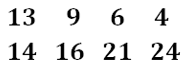(a) 16
(b) 20
(c) 27
(d) 17
(e) None of these

Q2. What will be the multiplication of resultant of both the rows?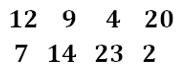(a) 184
(b) 188
(c) 186
(d) 183
(e) None of these

Q3. What is the sum of resultant of the both the rows?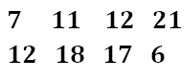(a) 38
(b) 36
(c) 40
(d) 46
(e) None of these

Q4. What will be the resultant of row I, if Y is the resultant of row II?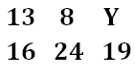(a) 12
(b) 13
(c) 11
(d) 15
(e) None of these

Directions (5-8): In every question two rows are given and to find out the resultant of a particular row you need to follow the given steps:
Step I: If an odd number is followed by an even number, then the resultant will be the multiplication of both numbers.
Step II: If an even number is followed by an odd number, then the resultant will be the addition of the both numbers.
Step III: If an odd number is followed by odd number, then the resultant will difference of the squares of both numbers.
Step IV: If an even number is followed by an even number, then the resultant will be the addition of the both numbers.

Q5. What is the difference of resultant of the both the rows?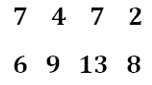(a) 6
(b) 8
(c) 10
(d) 9
(e) None of these

Q6. What is the addition of resultant of both the rows?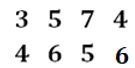(a) 175
(b) 182
(c) 180
(d) 176
(e) None of these

Q7. What is the half of the addition of resultant of both the rows?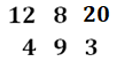(a) 110
(b) 112
(c) 100
(d) 105
(e) None of these

Q8. What will be the multiplication of resultant of both the rows?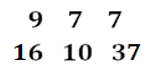(a) 2457
(b) 2456
(c) 2356
(d) 2458
(e) None of these

Directions (9-11): Answer the following question based on the information given below.
Eight stones (1, 2, 3, 4, 5, 6, 7 & 8) of different weights are placed in decreasing order of their weights from the left end. 2 is heavier than only 1 and 5. 6 is lighter than 8, which is not the heaviest stone. 7 and 6 are heavier than 3. 3 is heavier than 4. The second heaviest stone weighs 90 kg. There is a difference of 15 kg between the weight of stone 3 and 8.

Q9. What can be the possible weight of stone 6?(a) 60 kg
(b) 70 kg
(c) 80 kg
(d) 95 kg
(e) Can’t be determined

Q10. How many stones are heavier than stone 6?

(a) 2
(b) 3
(c) 4
(d) 5
(e) None of the above

Q11. How many stones are lighter than stone 7?

(a) 6
(b) 7
(c) 4
(d) 5
(e) None of the above

Directions (12-13): Answer the following question based on the information given below.
Seven kids (N, Z, K, R, J, T and L) have different ages. Age of N is 13 years. N is 4 years older than J. R is 2 years older than K. L is 3 years younger than K. L is 5 years old. The difference between ages of L and T is 10 years. Z is 4 years old.

Q12. Who is the eldest among all?

(a) N
(b) T
(c) J
(d) R
(e) None of the above

Q13. What is the age difference between N and L?
(a) 7
(b) 8
(c) 9
(d) 10
(e) None of the above

Directions (14-15): Answer the following question based on the information given below.
Six players (A, L, M, R, W and Z) have different number of balls. W has more balls than only two players. A has more balls than M and L but less ball than Z. R has more balls than A. L does not have lowest number of balls. R does not have highest number of balls.

Q14. How many players have lesser number of balls than L?
(a) None
(b) One
(c) Two
(d) Three
(e) None of the above

Q15. Who has highest number of balls?
(a) R
(b) Z
(c) A
(d) L
(e) None of the above

Solutions

Solution (1-4):
S1. Ans. (d)
Sol. In row 1, according to the given step, resultant of row 1 is 60.
In row 2, according to the given step, resultant of row 2 is 43.
Hence, required difference = 60 – 43 = 17.

S2. Ans. (a)
Sol. In row1, according to the given step, resultant of row 1 is 8.
In row 2, according to the given step, resultant of row 2 is 23.
Hence, required multiplication = 8 x 23 = 184.

S3. Ans. (b)
Sol. In row 1, according to the given step, resultant of row 1 is 19.
In row 2, according to the given step, resultant of row 2 is 17.
Hence, required sum = 19 + 17 = 36.

S4. Ans. (c)
Sol. In row 2, according to the given step, resultant of row 2 is 11. So, Y = 11
In row 1, according to the given step, resultant of row 1 is 11.

Solution (5-8):
S5. Ans. (a)
Sol. In row 1, according to the given step, resultant of row 1 is 70.
In row 2, according to the given step, resultant of row 2 is 64.
Hence, required difference = 70 – 64 = 6.

S6. Ans. (b)
Sol. In row 1, according to the given step, resultant of row 1 is 92.
In row 2, according to the given step, resultant of row 2 is 90.
Hence, required sum = 92 + 90 = 182.

S7. Ans. (c)
Sol. In row 1, according to the given step, resultant of row 1 is 40.
In row 2, according to the given step, resultant of row 2 is 160.
Hence, required half of the sum = 40 + 160 = 200/2 = 100.

S8. Ans. (a)
Sol. In row 1, according to the given step, resultant of row 1 is 39.
In row 2, according to the given step, resultant of row 2 is 63.
Hence, required multiplication = 39 x 63 = 2457.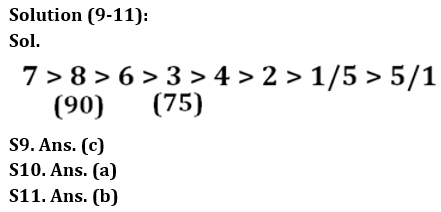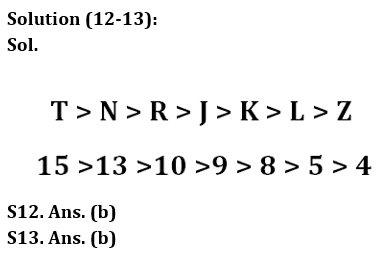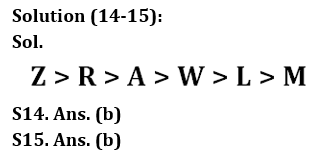## FAQs

### When will the LIC AAO Mains exam be held?

LIC AAO Mains exam will be held on 18 March

#### Congratulations!Union Budget 2023-24: Free PDF